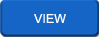## GraphTheory

 Basic Graph AlgorithmsAlgorithms for systematic searching,Strongly connected components,Strongly connected components Running times and further information
Rate this Book:
 Graph Theory Lecture NotesIntroduction,Definitions and Examples,Eulerian Graphs Hamiltonian Graphs,Graph Connectivity,Tree Graphs,Graph Planarity,Graph Embedding Graph Coloring
Rate this Book:
 Graph TheoryASix-Node Graph graph is a formal mathematical representation of a network ("a collection of objects connected in some fashion").
Rate this Book:
 Graph Theory GlossaryThis glossary is written to supplement the Interactive Tutorials in Graph Theory. Here we define the terms that we introduce in our tutorials--you may need to go to the library to find the definitions of more advanced terms. Please let me know of any corrections or suggestion!
Rate this Book:
 Graph MatrixesIsomorphism, Matrices and Graph Invariants; Subgraphs and Connectivity in Graphs
Rate this Book:
 Planarity BasicsPlanarity Basics are displayed in this pdf
Rate this Book:
 PlanarityInstructions: Arrange the vertices such that no edges overlap.
Rate this Book:
 Graph ConnectivityThere are many simple algorithms for Graph Connectivity problems, which makes it ideal for beginners.
Rate this Book:
 Vertex Descriptors and Graph ColoringThe problem of vertex partitioning in graphs can be solved in different ways ,. The most frequently used discriminating criteria are well-known square matrices that collect contributions of pairs of vertices from the graph
Rate this Book:
 TreesAn acyclic graph (also known as a forest) is a graph with no cycles. A tree is a connected acyclic graph. Thus each component of a forest is tree, and any tree is a connected forest.
Rate this Book:
 Bounded-Degree Spanning TreesGiven a complete graph with edge weights satisfying the triangle inequality,and a degree bound for each vertex, we consider the problem of computing a low-weight spanning tree in which the degree of each vertex is at most its given bound.
Rate this Book:
 Graph ColoringHow many colors did you use?,Two rules for coloring,Color each map Draw a map that requires 5 colors
Rate this Book:
 Planarity Testingst-Numbering, Bush Form and PQ-Tree, Vertex Addition Algorithm Finding Planar Embedding
Rate this Book:
 Planar GraphsA planar graph is one that can be drawn on a plane in such a way that there are no "edge crossings," i.e. edges intersect only at their common vertices.
Rate this Book:
 Planar GraphNote that while graph planarity is an inherent property of a graph, it is still possible to draw nonplanar embeddings of planar graphs. For example, the two embeddings above both correspond to the planar tetrahedral graph, but while the left embedding is planar, the right embedding is not. A planar embedding of a planar graph is sometimes called a plane graph (Harborth and Möller 1994).
Rate this Book:
 Introduction to Graph TheorySocial network data consists of binary social relations. That is, it records the presence, absence or strength of relationships among pairs of persons. There are many kinds of social relations
Rate this Book:
 Information SecurityInformation security is one of the cornerstones of the Information Society. Integrity of financial transactions, accountability for electronic signatures, confidentiality within a virtual enterprise, privacy of personal information
Rate this Book:
 Graph Theory IntroductionIn the branch of mathematics called Graph Theory, a graph bears no relation to the graphs that chart data, such as the progress of the stock market or the growing population of the planet. Graph paper is not particularly useful for drawing the graphs of Graph Theory.
Rate this Book:
 Graphs and ConnectivityYou are undoubtedly familiar with graphs of functions from your experience in algebra courses: y = f(x) on the Cartesian Plane. Those graphs all shared one common characteristic: any two graphs which appeared to be different did indeed represent different functions (as long as the units on the coordinate axes were the same).
Rate this Book:
 Graphs and MatricesA binary relation R is a set of ordered pairs (x,y). Each element in an ordered pair is drawn from a (potentially different) set. The ordered pairs relate the two sets: together, they comprise a mapping, which is another name for a relation.
Rate this Book:
 Graph PlanarityA graph G is planar if it can be drawn in the plane in such a way that no two edges meet each other except at a vertex to which they are incident. Any such drawing is called a plane drawing of G.
Rate this Book:
 A directed GraphSometimes a digraph is called a simple digraph to distinguish it from a directed multigraph, in which the arcs constitute a multiset, rather than a set, of ordered pairs of vertices. Also, in a simple digraph loops are disallowed. (A loop is an arc that pairs a vertex to itself.) On the other hand, some texts allow loops, multiple arcs, or both in a digraph.
Rate this Book:
 Dijkstra's Shortest Path AlgorithmIn recitation we talked a bit about graphs: how to represent them and how to traverse them. Today we will discuss one of the most important graph algorithms: Dijkstra's shortest path algorithm, a greedy algorithm that efficiently finds shortest paths in a graph. (Pronunciation: "Dijkstra" is Dutch and starts out like "dike").
Rate this Book: